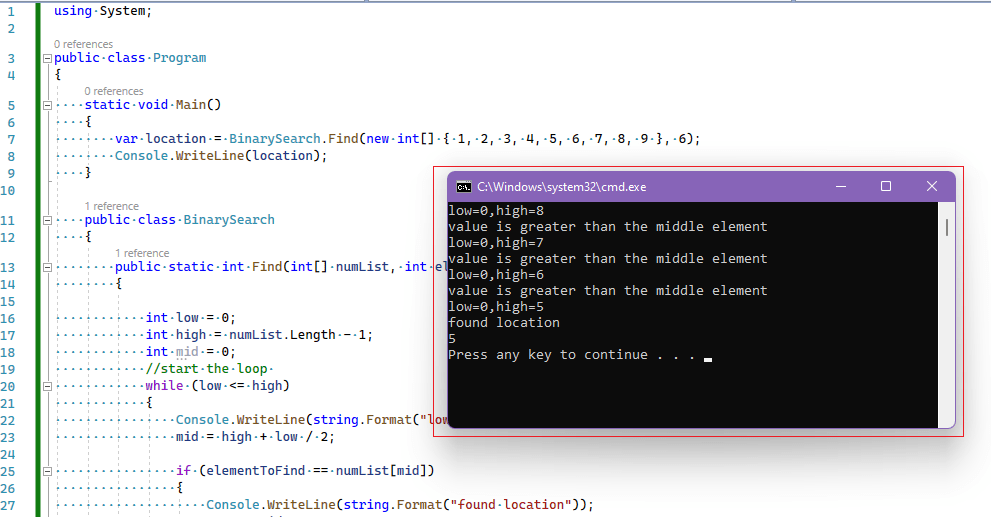In previous article, I mentioned Armstrong number in C# (Console Application Program) but in this article, I have mentioned what is binary search with C# example in Console application.

Binary Search is one of the methods of searching an item in a list of items

In Binary search, we first need to calculate the middle element in the list and then compare the element we are searching with this middle element.

To calculate middle element we use the formula:

``middle element = highest element + lowest element / 2``

After we calculate the middle element we compare the element we are searching for with this element.

There can be three conditions:

• if value is equal to the middle element, then we found the item
• if value is less than the middle element, then we check remaining greater value items in list
• if value is greater than the middle element, then we check remaining less value items in list

So, we keep on repeating above conditions until we find the value.

``````using System;
using System.Text;

public class Program
{
static void Main() {
var location = BinarySearch.Find(new int[] {1,2,3,4,5, 6, 7,8,9}, 6);
Console.WriteLine(location);
}

public class BinarySearch {
public static int Find(int[] numList, int elementToFind) {

int low = 0;
int high = numList.Length - 1;
int mid = 0;
//start the loop
while (low <= high) {
Console.WriteLine(string.Format("low=0,high={1}", low, high));
mid = high + low / 2;

if (elementToFind == numList[mid]) {
Console.WriteLine(string.Format("found location"));
return mid;
}
if (elementToFind > numList[mid]) {
Console.WriteLine(string.Format("value is less than the middle element"));
low = mid + 1;
Console.WriteLine(string.Format("low=0,high={1},mid={2}", low, high, mid));
} else {
Console.WriteLine(string.Format("value is greater than the middle element"));
high = mid - 1;
}

}

return -1;

}
}

}
``````

Output:

``````low=0,high=8
value is greater than the middle element
low=0,high=7
value is greater than the middle element
low=0,high=6
value is greater than the middle element
low=0,high=5
found location
5``````### Where is Binary search used?

• In libraries of Java, .Net, C++ STL
• While debugging, the binary search is used to pinpoint the place where the error happens.

That's it, hope it helped.

You may also like to read:

Reverse string program in C# (Various methods)

Armstrong number in C# (Console Application Program)